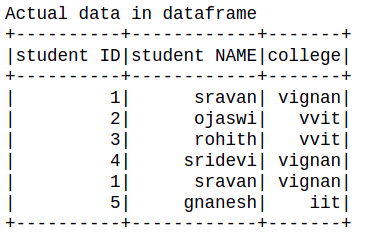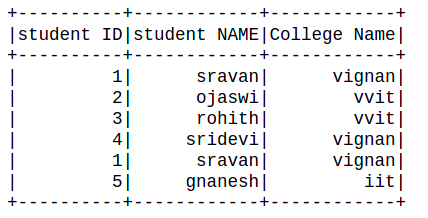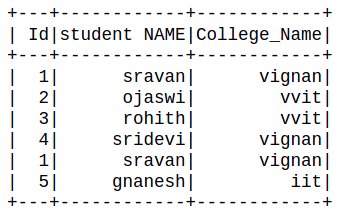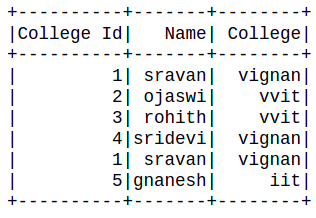# How to rename multiple columns in PySpark dataframe ?

• Last Updated : 04 Jul, 2021

In this article, we are going to see how to rename multiple columns in PySpark Dataframe.

Before starting let’s create a dataframe using pyspark:

Attention geek! Strengthen your foundations with the Python Programming Foundation Course and learn the basics.

To begin with, your interview preparations Enhance your Data Structures concepts with the Python DS Course. And to begin with your Machine Learning Journey, join the Machine Learning - Basic Level Course

## Python3

 `# importing module``import` `pyspark``from` `pyspark.sql.functions ``import` `col`` ` `# importing sparksession from pyspark.sql module``from` `pyspark.sql ``import` `SparkSession`` ` `# creating sparksession and giving an app name``spark ``=` `SparkSession.builder.appName(``'sparkdf'``).getOrCreate()`` ` `# list  of students  data``data ``=` `[[``"1"``, ``"sravan"``, ``"vignan"``],``        ``[``"2"``, ``"ojaswi"``, ``"vvit"``],``        ``[``"3"``, ``"rohith"``, ``"vvit"``],``        ``[``"4"``, ``"sridevi"``, ``"vignan"``],``        ``[``"1"``, ``"sravan"``, ``"vignan"``],``        ``[``"5"``, ``"gnanesh"``, ``"iit"``]]`` ` `# specify column names``columns ``=` `[``'student ID'``, ``'student NAME'``, ``'college'``]`` ` `# creating a dataframe from the lists of data``dataframe ``=` `spark.createDataFrame(data, columns)`` ` `print``(``"Actual data in dataframe"``)`` ` `# show dataframe``dataframe.show()`

Output:Method 1: Using withColumnRenamed.

Here we will use withColumnRenamed() to rename the existing columns name.

Syntax: withColumnRenamed( Existing_col, New_col)

Parameters:

• Existing_col: Old column name.
• New_col: New column name.

Example 1: Renaming single columns.

## Python3

 `dataframe.withColumnRenamed(``"college"``, ``                            ``"College Name"``).show()`

Output:Example 2: Renaming multiple columns.

## Python3

 `df2 ``=` `dataframe.withColumnRenamed(``"student ID"``,``                                  ``"Id"``).withColumnRenamed(``"college"``,``                                                          ``"College_Name"``)``df2.show()`

Output:Method 2: Using toDF()

This function returns a new DataFrame that with new specified column names.

Syntax: toDF(*col)

Where, col is a new column name

In this example, we will create an order list of new column names and pass it into toDF function.

## Python3

 `Data_list ``=` `[``"College Id"``,``" Name"``,``" College"``]``new_df ``=` `dataframe.toDF(``*``Data_list)``new_df.show()`

Output:My Personal Notes arrow_drop_up